# Optical Character Recognition using Logistic Regression (Linear Models)

Visual understanding enables learning more than any other form of learning. We see! We analyze! And We learn! Following the pathway of humans, now Machine Learning models can also recognize the characters present in images. The technique of recognizing characters using machine learning or computer vision has become very popular among industries. Google, Microsoft, Twitter, Mathworks, and many more industrial giants are using OCR techniques to solve a wide variety of tasks, including spam classification, automatic reply, or number-plate detection.

#### Key takeaways from this blog

1. What is a character recognition task?
2. Gist about the MNIST data.
3. Logistic regression in action.
4. Steps to implement the logistic regression.
5. Company use-case for this machine learning application.
6. Possible interview questions on this project.

Character recognition is a primary step in recognizing whether any text or character is present and whether our algorithms can recognize it or not in the given image. From understanding a text to identifying an object in an image to scene understanding, everything relies on the basic task of recognition. To understand it clearly, let’s take an example.

In the image shown below, character recognition can solve simple problems such as identifying the characters in the number plate. This recognition can be mapped to data association problems such as identifying the vehicle with a particular ID and check whether it is present at a certain location or violated any traffic law.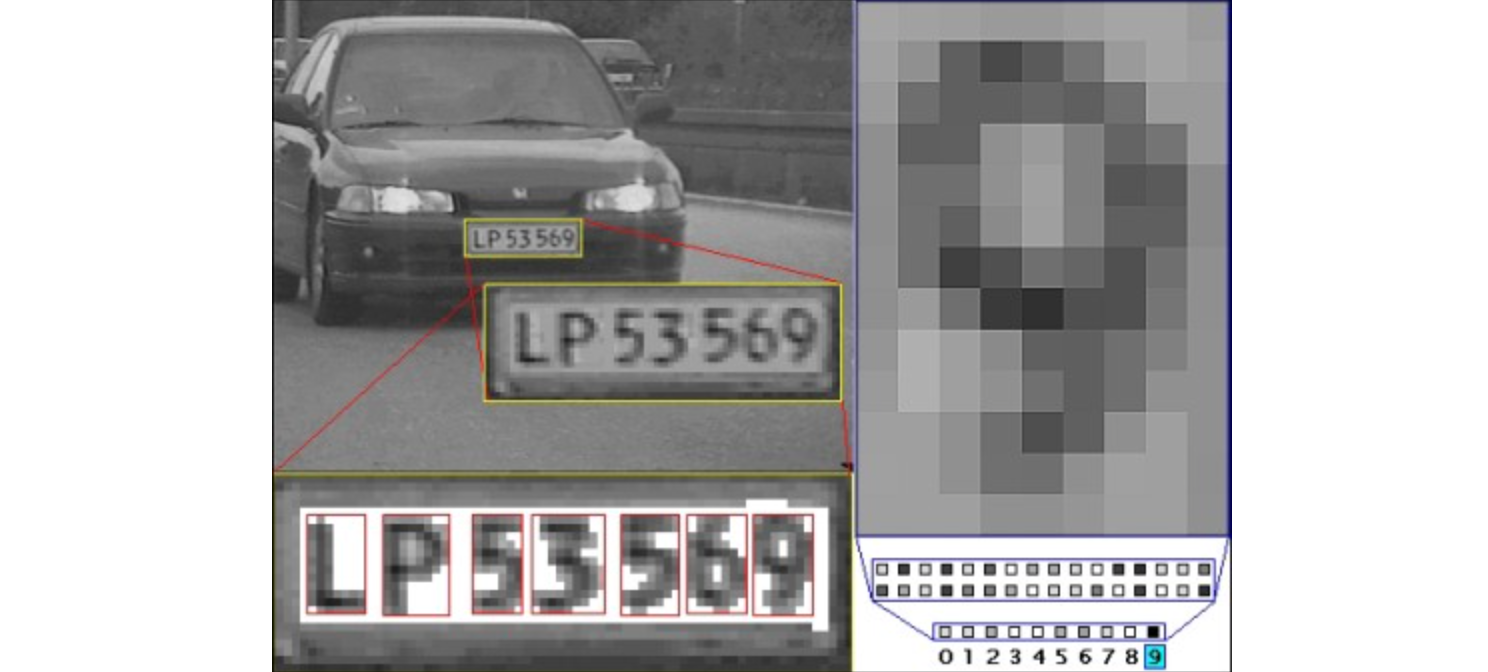Character Recognition

We all know what deep learning has accomplished so far. Many computer vision applications that were supposed to be impossible few years back are now possible due to deep learning. It has attained a state-of-the-art in problems like OCR. Many deep-learning architectures are developed over the years, focusing on the problems discussed above. Indeed, we need a deeper neural network to solve complex tasks, but for certain simple image classification problems, a linear model can do a decent job for us. The good thing with linear models are,

1. They are computationally cheap and hence can be easily deployed on IoT devices.
2. They are efficient in terms of time and provide predictions in real-time.

The problem of simple character recognition can be solved using algorithms like Multi-Layer Perceptron (MLP), SVMs, Logistic Regression, etc.
In this article, we will describe the steps to implement a Logistic Regression classifier for character recognition. So let’s start without any further delay.

### Dataset description

We will use the MNIST dataset. The MNIST dataset is a 28x28 pixel² sized open-source dataset commonly available in scikit-learn and other machine-learning frameworks.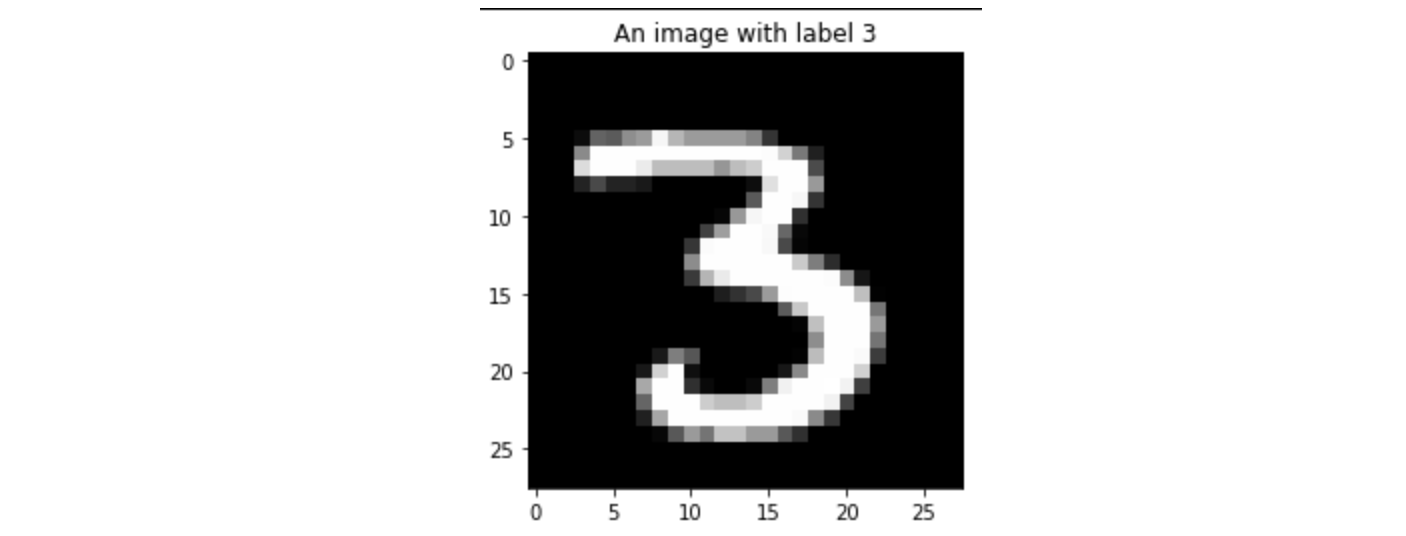A sample image from the MNIST dataset

The above image is a sample from the MNIST handwritten digit dataset. We will use such samples to show how a linear model can perform the task of recognizing hand-written digits
For cases where the image is well enhanced (In a human-readable format), no form of restoration (cleaning of images) is needed, and no need for any data augmentation (transformation of images) or domain adaptation (when train and test lie in different distributions) of the input image features is required. A linear model can deliver decent accuracy.

### Steps to implement

#### Dataset

We will be using the sklearn library to import the data. The MNIST dataset has images of size 28x28 which, when flattened out, will give a vector 784x1. Use the fetch_openml package from the sklearn.dataset and import the flattened vector dataset using ‘mnist_784’. This dataset will be used for the Logistic Regression classifier model.

``````X, y = fetch_openml('mnist_784', version=1,
return_X_y=True, as_frame=False)
``````

#### Building a Logistic Regression:

The MNIST dataset has images of size 28x28, and we are going to flatten that out to provide our regression classifier an input format on which it can be trained. Logistic Regression falls in the category of Generalized Linear Models (GLM) and is very much like linear regression, except logistic regression predicts categorical target variables instead of continuous data. This means that the final layer output values in logistic regression are probability values between 0 and 1, which classify any observation into a particular category. The model can be imported from sklearn.linear_model with tunable parameters such as the strength of regularization, penalty type.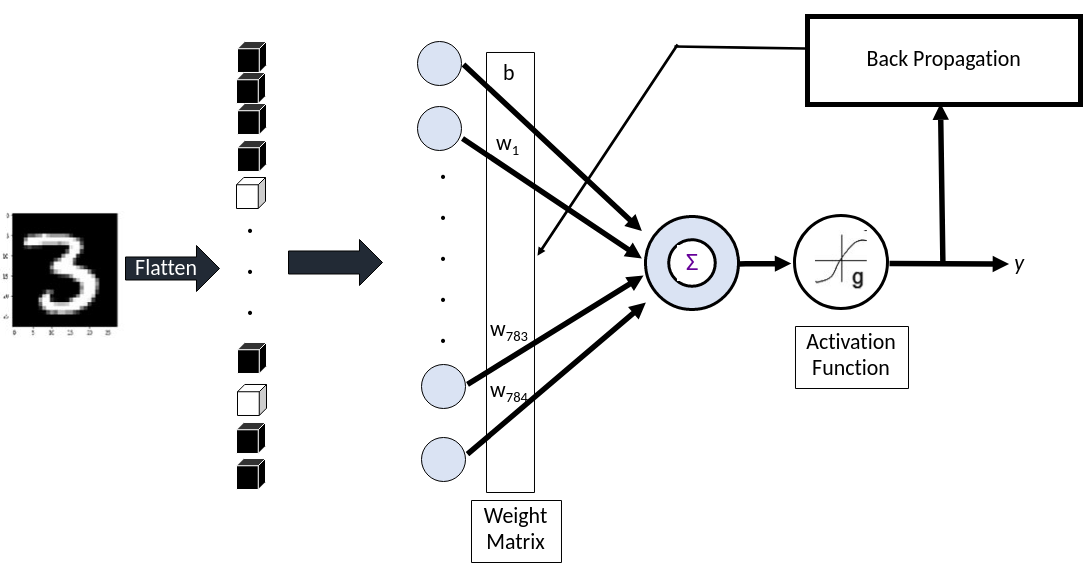#### Model Fitting

Three major steps that can be filled are,

1. Split the data (say) 70:30 for train and test.
2. Create an instance of the Logistic Regression model and fit it on the train data.
clf = LogisticRegression(C=50. / train_samples, penalty=’l1', solver=’saga’, tol=0.1)
3. Vary the model hyper-parameters from low to high strength of regularization (C:[5e-4:500]) and for a different type of scaling-based preprocessing.

#### Analyze the model prediction:

First, we will see how the model has performed under different scenarios. We will be using different combinations by varying the pre-processing types and the strength of regularization. To evaluate the model, we will be using the accuracy parameter.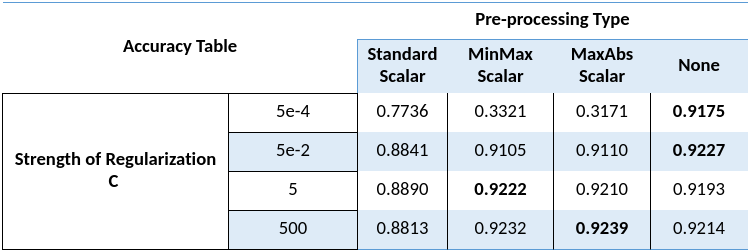#### Observations

1. Scalar pre-processing need not always help a model develop its generalization ability. It is clearly evident that without pre-processing, the model was performing well.
2. Tuning a model to hard regularization can aid the model in improving its generalization.

#### Model weight visualization

Let us view how the weight matrix corresponding to each output class looks like.

1. Collect one sample of each category of the MNIST dataset.
2. The model weights are reshaped to view(28,28) to plot like the images below.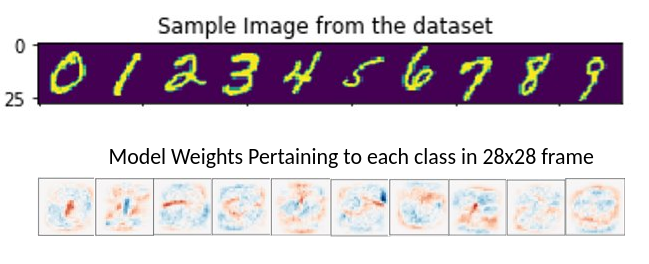When plotted out, the weight matrix shows how the model has learned its parameters to provide its decision while predicting a class.

### Company Use-case

Character recognition has become an integral part of computer vision and analysis, and as such several corporations are actively working to improve upon the current state-of-the-art. Several industries are actively working on developing algorithms that can precisely categorize hand-written digits and notes. Tools such as Optical Character Recognition (OCR) are in practice now and have achieved the state-of-the-art in such objectives.

Cloud Vision lets algorithm designers integrate optical character recognition (OCR ), vision detection features, including image labeling. These algorithms can be easily incorporated into the user’s overall pipeline. Check out the documentation to learn how to use such APIs and the overall package of services offered by them.#### Computer Vision API, Microsoft Azure

Microsoft’s Azure’s Computer Vision API includes Optical Character Recognition (OCR) capabilities that extract printed or handwritten text from images. This API provides developers with access to advanced image processing algorithms that have attained the current state-of-the-art. This API works in several languages, making it one of the most used characters recognition API.### Possible Interview Questions

Based on this project, interviewers can ask these questions in your machine learning interview:

1. What is logistic regression? Whether it is a classification algorithm or regression algorithm?
2. How are linear models better to solve lesser complex problems?
3. What is the cost function associated with the linear regression?
4. What are the hyperparameters that need to be tuned to achieve better accuracy?
5. What are generalized linear models?
6. Did you use some data pre-processing things on images? What is data augmentation, and what kind of preprocessing is required for image data?

### Conclusion

In this article, we discussed one of the best machine learning applications: optical character recognition. We discussed the steps to implement a linear classifier model over the MNIST dataset and evaluated the performance. After that, we discussed some of the use-cases of companies that are currently using this technology. We hope you have enjoyed the article and sensed how efficient even the linear machine learning models can be.

#### Enjoy Learning! Enjoy Thinking!

Get well-designed application and interview centirc content on ds-algorithms, machine learning, system design and oops. Content will be delivered weekly.